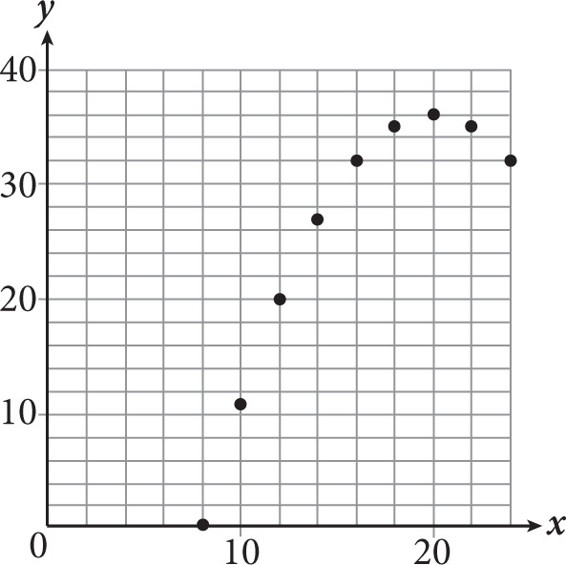# SAT Math Multiple Choice Question 419: Answer and Explanation

### Test Information

Question: 419

14.If a quadratic equation is used to model the data shown in the scatterplot above, and the model fits the data exactly, which of the following is a solution to the quadratic equation?

• A. 28
• B. 32
• C. 34
• D. 36